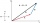# 3d vector component

The vector u = (3.9, u3) and the length of the vector u is 12. What is is u3?

Result

u3 =  7.348
u3 =  -7.348

#### Solution:Checkout calculation with our calculator of quadratic equations.

Leave us a comment of example and its solution (i.e. if it is still somewhat unclear...):Be the first to comment!#### To solve this example are needed these knowledge from mathematics:

For Basic calculations in analytic geometry is helpful line slope calculator. From coordinates of two points in the plane it calculate slope, normal and parametric line equation(s), slope, directional angle, direction vector, the length of segment, intersections the coordinate axes etc. Looking for help with calculating roots of a quadratic equation? Two vectors given by its magnitudes and by included angle can be added by our vector sum calculator. Pythagorean theorem is the base for the right triangle calculator.

## Next similar examples:Given that P = (5, 8) and Q = (6, 9), find the component form and magnitude of vector PQ.
2. Unit vector 2DDetermine coordinates of unit vector to vector AB if A[-6; 8], B[-18; 10].
3. Vector 7Given vector OA(12,16) and vector OB(4,1). Find vector AB and vector |A|.
4. Vector - basic operationsThere are given points A [-9; -2] B [2; 16] C [16; -2] and D [12; 18] a. Determine the coordinates of the vectors u=AB v=CD s=DB b. Calculate the sum of the vectors u + v c. Calculate difference of vectors u-v d. Determine the coordinates of the vector w.
5. Coordinates of vectorDetermine the coordinate of a vector u=CD if C(19;-7) and D(-16;-5)
6. Triangle IRTIn isosceles right triangle ABC with right angle at vertex C is coordinates: A (-1, 2); C (-5, -2) Calculate the length of segment AB.
7. Three pointsThree points A (-3;-5) B (9;-10) and C (2;k) . AB=AC What is value of k?
8. Theorem proveWe want to prove the sentence: If the natural number n is divisible by six, then n is divisible by three. From what assumption we started?
9. ProductThe product of two consecutive odd numbers is 8463. What are this numbers?
10. DistanceWha is the distance between the origin and the point (18; 22)?
11. RootsDetermine the quadratic equation absolute coefficient q, that the equation has a real double root and the root x calculate: ?Find the roots of the quadratic equation: 3x2-4x + (-4) = 0.Determine the discriminant of the equation: ?Equation ? has one root x1 = 8. Determine the coefficient b and the second root x2.Solve quadratic equation: 2x2-58x+396=0How much is sum of square root of six and the square root of 225?Number ?. Find the value of x.